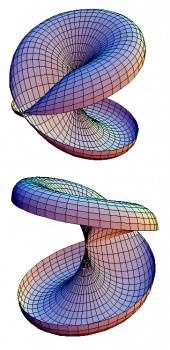Algebraic, Computational and Differential Geometry

The research interests of the group concern algebraic, computational, differential and topological aspects of geometry. Topics of individual group members include: the study of algebraic varieties from both the intrinsic and embedded viewpoint; parameter spaces of low dimensional algebraic varieties (moduli spaces of curves and surfaces, Hilbert schemes of points and curves, Severi varieties); low codimensional projective subvarieties and vector bundles; intersection theory on Grassmannians and flag varieties; computational methods in commutative algebra and algebraic geometry; discrete differential geometry; Lie groups and algebras; Riemannian varieties with special holonomy; Kähler and symplectic geometry; descriptive dynamics and bifurcation theory; set theory and mathematical logic; topology of the real line and measure theory; analytic, algebraic and arithmetic methods in number theory.